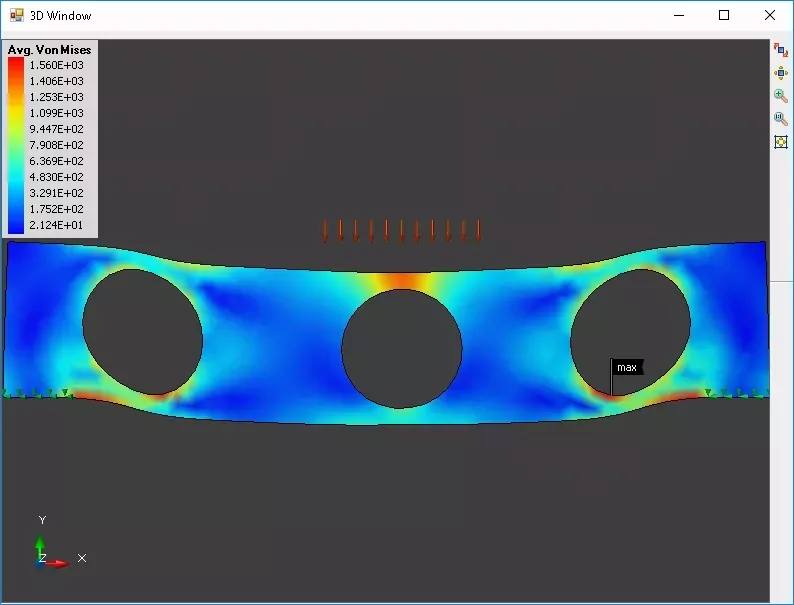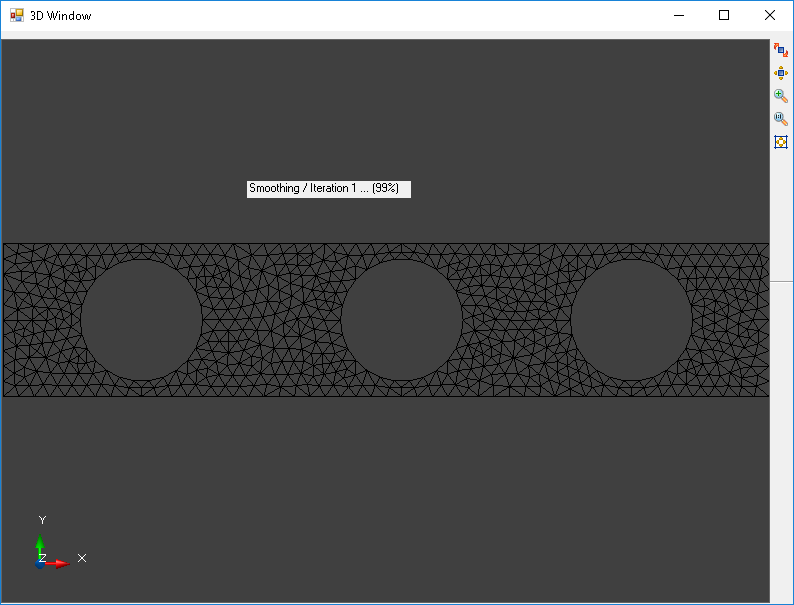.Net Library for Finite Element Analysis

Finite element analysis is a really cool technique that can be applied to solve lots of engineering problems. Imagine that you’re job is to model how a structural part will react when a force is applied to it. Within the material there could be trillions of atoms that are interacting with each other, some pushing and some pulling one another, how would you begin to compute those areas that are high stress? That is what finite element analysis does for us, we can break down the impossibly large problem into smaller pieces by meshing the physical structure and then computing the interactions of each node in our mesh, think of it as eating the elephant one byte at a time.Meshing

Meshing is performed on the material structure in such a way that each of the elements is a relatively uniform size.

To setup our test we create a bar that has three large holes in it.

var mesher = new BasicMesher()
.Build(true, 1))
.Build(true, 1))
.Build(true, 1))
Next, the mesher is invoked on the input shapes outline. After several passes of refinement a mesh of acceptable quality is produced. The result of the mesh generation looks like this